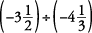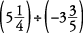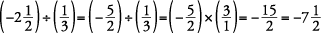## Rationals (Signed Numbers Including Fractions)

Recall that integers are positive and negative whole numbers and zero. When fractions and terminating or repeating decimals between the integers are included, the complete group of numbers is referred to as rational numbers. They are signed numbers including fractions. A more technical definition of a rational number is any number that can be written as a fraction with the numerator being a whole number or integer and the denominator being a natural number. Notice that fractions can be placed on the number line, as shown in Figure 1

Fractions may be negative as well as positive. Negative fractions are typically written as follows: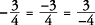Although they are all equal.

The rules for signs when adding integers applies to fractions as well. Remember: To add fractions, you must first get a common denominator.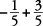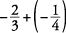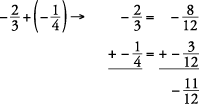The rules for signs when adding integers applies to mixed numbers as well.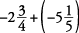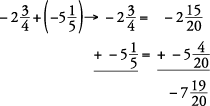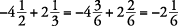The rules for signs when subtracting integers applies to fractions as well. Remember: To subtract fractions, you must first get a common denominator.

Subtract the following.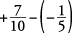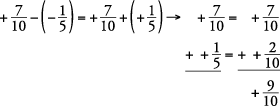The rules for signs when subtracting integers applies to mixed numbers as well. Remember: To subtract mixed numbers, you must first get a common denominator. If borrowing from a column is necessary, be cautious of simple mistakes.

Subtract the following.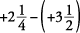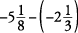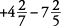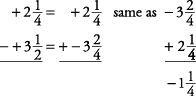Problems, such as the preceding ones, are usually most easily done by stacking the number with the larger absolute value on top, subtracting, and keeping the sign of the number with the larger absolute value.

The rules for signs when multiplying integers applies to fractions as well. Remember: To multiply fractions, multiply the numerators and then multiply the denominators. Always simplify to lowest terms if possible.

Multiply the following.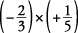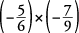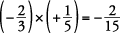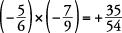You can cancel when multiplying positive and negative fractions. Simply cancel as you do when multiplying positive fractions, but pay special attention to the signs involved. Follow the rules for signs when multiplying integers to obtain the proper sign. Remember: No sign means that a positive sign is understood.

Multiply the following.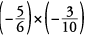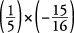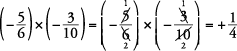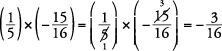Follow the rules for signs when multiplying integers to get the proper sign. Remember: Before multiplying mixed numbers, you must first change them to improper fractions.

Multiply the following.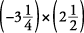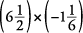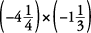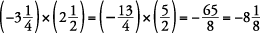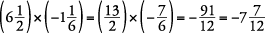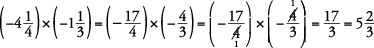Follow the rules for signs when dividing integers to get the proper sign. Remember: When dividing fractions, first invert the divisor and then multiply.

Divide the following.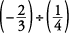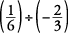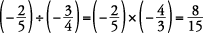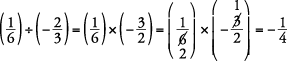Follow the rules for signs when dividing integers to get the proper sign. Remember: Before dividing mixed numbers, you must first change them to improper fractions. Then you must invert the divisor and multiply.

Divide the following.